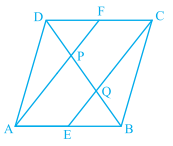"
">

# In a parallelogram $\mathrm{ABCD}, \mathrm{E}$ and $\mathrm{F}$ are the mid-points of sides $\mathrm{AB}$ and $\mathrm{CD}$ respectively (see below figure). Show that the line segments AF and $\mathrm{EC}$ trisect the diagonal BD."

Given:

In a parallelogram $\mathrm{ABCD}, \mathrm{E}$ and $\mathrm{F}$ are the mid-points of sides $\mathrm{AB}$ and $\mathrm{CD}$ respectively.

To do:
We have to show that the line segments $AF$ and $\mathrm{EC}$ trisect the diagonal $BD$.

Solution:

$A B C D$ is a parallelogram.

We know that,

Opposite sides of a parallelogram are equal and parallel.

This implies,

$A B \| D C$

$A B=D C$

$\Rightarrow A E \| F C$

$\frac{1}{2} A B=\frac{1}{2} D C$

$\Rightarrow \mathrm{AE} \| \mathrm{FC}$

$\mathrm{AE}=\mathrm{FC}$

Therefore,

$AECF$ is a parallelogram.

This implies,

$\mathrm{AF} \| \mathrm{EC}$

$\Rightarrow \mathrm{EQ} \| \mathrm{AP}$ and $\mathrm{FP} \| \mathrm{CQ}$

In $\triangle B A P$,

$E$ is the mid-point of $A B$ and $E Q \| A P$.

By converse of mid-point theorem,

$Q$ is the mid-point of $B P$.

This implies,

$\mathrm{BQ}=\mathrm{PQ}$...........(i)

In $\triangle D Q C$,

$F$ is the mid-point of $D C$

$F P \| C Q$

By converse of mid-point theorem,

$P$ is the mid-point of $D Q$.

Therefore,

$\mathrm{PQ}=\mathrm{DP}$........(ii)

From (i) and (ii), we get,

$B Q=P Q=P D$

Therefore, $CE$ and $AF$ trisect the diagonal $BD$.

Updated on: 10-Oct-2022

19 Views